# Mahotas – Dilating Image

In this article we will see how we can dilate the image in mahotas. Dilation adds pixels to the boundaries of objects in an image, while erosion removes pixels on object boundaries. The number of pixels added or removed from the objects in an image depends on the size and shape of the structuring element used to process the image.

In this tutorial we will use “luispedro” image, below is the command to load it.

`mahotas.demos.load('luispedro')`

Below is the luispedro image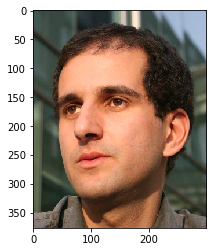In order to do this we will use `mahotas.morph.dilate`method

Syntax : mahotas.morph.dilate(image)

Argument :It takes image object as argument

Return : It returns image object

Note : Input image should be filtered or should be loaded as grey

In order to filter the image we will take the image object which is numpy.ndarray and filter it with the help of indexing, below is the command to do this

`image = image[:, :, 0]`

Below is the implementation

 `# importing required libraries ` `import` `mahotas ` `import` `mahotas.demos ` `from` `pylab ``import` `gray, imshow, show ` `import` `numpy as np ` ` `  `# loading iamge ` `luispedro ``=` `mahotas.demos.load(``'luispedro'``) ` ` `  `# filtering image ` `luispedro ``=` `luispedro.``max``(``2``) ` ` `  `# otsu method ` `T_otsu ``=` `mahotas.otsu(luispedro) ` `  `  ` `  `# image values should be greater than otsu value ` `img ``=` `luispedro > T_otsu ` ` `  `print``(``"Image threshold using Otsu Mehtod"``) ` ` `  `# showing image ` `imshow(img) ` `show() ` ` `  `# dilating image ` `dilate_img ``=` `mahotas.morph.dilate(img) ` ` `  `# showing dilated image ` `print``(``"Dilated Image"``) ` `imshow(dilate_img) ` `show() `

Output :

`Image threshold using Otsu Mehtod`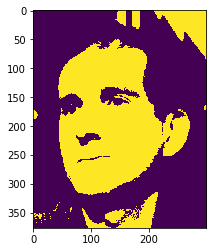`Dilated Image`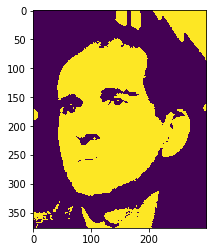Another example

 `# importing required libraries ` `import` `mahotas ` `import` `numpy as np ` `import` `matplotlib.pyplot as plt ` `import` `os ` `  `  `# loading iamge ` `img ``=` `mahotas.imread(``'dog_image.png'``) ` `       `  `# setting filter to the image ` `img ``=` `img[:, :, ``0``] ` ` `  `# otsu method ` `T_otsu ``=` `mahotas.otsu(img) ` `  `  ` `  `# image values should be greater than otsu value ` `img ``=` `img > T_otsu ` ` `  `print``(``"Image threshold using Otsu Mehtod"``) ` ` `  `# showing image ` `imshow(img) ` `show() ` ` `  `# dilating image ` `dilate_img ``=` `mahotas.morph.dilate(img) ` ` `  `# showing dilated image ` `print``(``"Dilated Image"``) ` `imshow(dilate_img) ` `show() `

Output :

`Image threshold using Otsu Mehtod`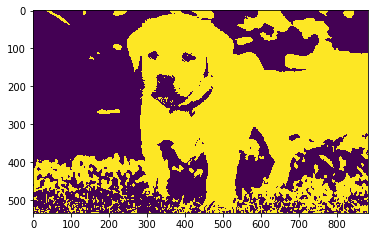`Dilated Image`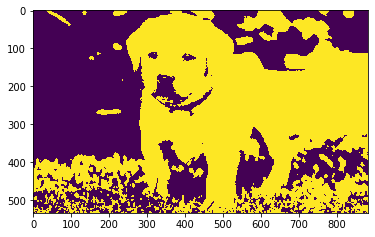My Personal Notes arrow_drop_upCheck out this Author's contributed articles.

If you like GeeksforGeeks and would like to contribute, you can also write an article using contribute.geeksforgeeks.org or mail your article to contribute@geeksforgeeks.org. See your article appearing on the GeeksforGeeks main page and help other Geeks.

Please Improve this article if you find anything incorrect by clicking on the "Improve Article" button below.

Article Tags :

Be the First to upvote.

Please write to us at contribute@geeksforgeeks.org to report any issue with the above content.